>Next: (ii) Graphs of Rsequence Up: (a) Calculation of Rsequence Previous: (a) Calculation of Rsequence

### (i) Formula for Rsequence

Data for calculating Rsequence comes from two sources. One is the nucleotide sequences at which a recognizer has been shown to bind. The other is the nucleotide composition of the genome in which the recognizer functions. The sequences are aligned by one base (the zero base) to give the largest possible homology between them (see figure 9 for an example). Some positions have little variation, while others have more. We tabulate the frequency of each base B at each position L in the site, to make a table called f(B,L). Focusing on one position at a time, we want to measure the possible variations. For this we have chosen the "uncertainty" measure introduced by Shannon in 1948 (Shannon, 1948; Shannon and Weaver, 1949; Weaver, 1949; Abramson, 1963; Singh, 1966; Gatlin, 1972; Sampson, 1976; Pierce, 1980; Campbell, 1982; Schneider, 1984).

When there are M possible symbols, with probabilities Pi (such that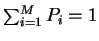) , the general formula for uncertainty is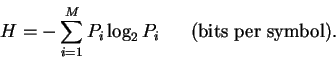(1)

One bit of information resolves the uncertainty of choice between two equally likely symbols. For nucleotide sequences, there are M=4 possible bases. Using the frequencies of bases as estimates for probabilities, the uncertainty is calculated as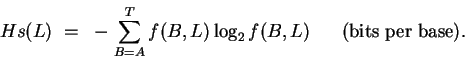(2)

(B is either A, C, G or T). The formula gives sensible results for three simple cases: 1) If only one base appears in the sequences, such as an A, then f(A,L)=1 while the other frequencies are zero. Hs(L) gives zero bits ( log 0 = 0), meaning that if we were to sequence another site, we would have no uncertainty that the next base will be an A. 2) If two bases appeared with equal frequency, [as in f(A,L)=0.5, f(C,L)=0, f(G,L)=0.5 and f(T,L)=0], our uncertainty would be 1 bit. 3) If all 4 bases appeared with equal frequencies, then f(B,L)=0.25 and the uncertainty is 2 bits.

If we sequenced randomly in the genome, and aligned sequences arbitrarily, we would see all 4 bases, with probabilities P(B) and our uncertainty about what base we would see next would be: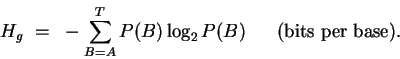(3)

This number is close to 2 bits for the organism E. coli, considered in this paper. In contrast, when sequences are aligned at binding sites (as in typical consensus alignments) a pattern appears which decreases the uncertainty below that of randomly aligned fragments (equation (2)). For each position L the decrease would be: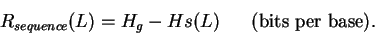(4)

This is a measure of the sequence information gained by aligning the sites. The total information gained will be the total decrease in uncertainty: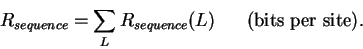(5)

(By summing, we make the simplifying assumption that the frequencies at one position are not influenced by those at another position. It is also possible to calculate Rsequencefrom dinucleotides or oligonucleotides [Shannon, 1951; Gatlin, 1972; Lipman and Maizel, 1982]. When dinucleotides were used for ribosome binding sites, the total information content was not different from that given in Results, [unpublished observation]. Unfortunately, sampling error prevents one from making the calculation in most cases.)Next: (ii) Graphs of Rsequence Up: (a) Calculation of Rsequence Previous: (a) Calculation of Rsequence
Tom Schneider
2002-10-16